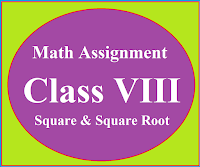### Math Assignment Class VIII | Square & Square RootMath Assignment  Class VIII | Square & Square Root Download or Print free  assignment with answer key  for   Class  8 Squares and  Square Roots.   Important and extra questions that cover all topics of square and square root and is useful and helpful for the students. Math Assignment  Class VIII | Square & Square Root LEVEL -1

### Math Assignment Class XI Ch-13 | Limits

Math Assignment  Class XI | Chapter 13 | Limits
Math Assignment  Class XI  Chapter 13  Limits and derivatives, Extra questions on Limits strictly according to the CBSE & DAV Board, Important questions on limits.

MATHS ASSIGNMENT ON LIMIT

Question 1 :

Evaluate the  limits:
Ans: -1/4

Question 2 :
Evaluate the  limits:
Ans: - 1/2

Question 3:
Evaluate the  limits
Ans:  -1

Question 4 :
Evaluate the  limits
Ans: 0

Question 5:
Evaluate the  limits
Ans: 4

Question 6 :
Evaluate the  limits
Ans: 9

Question 7 :

If f(x) =         then find

Ans: 2
Solution Hint:  Find LHL and RHL

Question 8:
Evaluate the  limits:
Ans: 3
Solution Hint:
Use formula:

Question 9 :

If    , then find the value of K

Ans:  3/7

Question 10 :
Evaluate the  limits:
Ans: 9/2

Question 11 :

If     then find the value of k

Ans: 8/3

Question 12 :
Evaluate the  limits:
Ans: 10

Question 13 :
Evaluate the  limits:
Ans: 8

Question 14 :
Evaluate the  limits:
Ans:

Question 15 :
Evaluate the  limits:
Ans: 1/2

Question 16 :
Evaluate the  limits:
Ans: 1/2

Question 17 :
Evaluate the  limits:
Ans: 3/16

Question 18 :
Evaluate the  limits
Ans: 3/4

Question 19 :
Evaluate the  limits

Ans: 1/2

Solution Hint:

Rationalizing the numerator.

Simplify and then putting the limit.

Question 20
Ans: 4/3

Question 21
Evaluate the  limits:
Ans: 1/2

Question 22
Evaluate the  limits:
Ans: - 4

Solution Hint

Use  Sin3x = 3sinx – 4sin3x,

putting  x = π+h, as x→ π, then h→0

Question 23
Evaluate the  limits:

Ans: 2/5
Solution Hint:       =

=     = 2/5

Question 24
Evaluate the  limits:
Ans: 1

Question 25
Evaluate the  limits:
Ans: - √2

Solution Hint:
=

=

=

=

=   =

Question 26
Evaluate the  limits:
Ans: 2
Solution Hint:

=

=

=

=2 x 1 = 2

Question 27
Evaluate the  limits:
Ans: Log5.Log2
Solution Hint:

=

=

=

=

=  Log5.Log2
Formula used :

Question 28
Evaluate the  limits:
Ans: -5/16
Solution Hint:

=

=

=

=

=     =

Question 29
Evaluate the  limits:

Ans: - 4

Solution Hint

Question 30
Evaluate the  limits:

Ans: 2/3